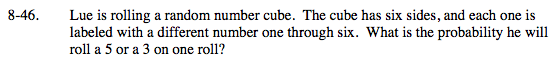### Home > MC2 > Chapter 8 > Lesson 8.2.2 > Problem8-46

8-46.Each number has an equal probability of being rolled. Since there are 6 total sides, what is the probability of getting any number?

Each number has a one-in-six probability of being rolled. Since we can roll either a 5 or a 3, we can add the probabilities of these two numbers together.

$\frac{1}{6} + \frac{1}{6} = ?$

Use the eTool to find the probability of rolling a 5 or a 3.
Click the link at right for the full version of the eTool: MC2 8-46 HW eTool